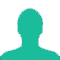# If the percentage impedances of the two transformers working in parallel are different, then

A. transformers will be overheated

B. power factors of both the transformers will be same

C. parallel operation will be not possible

D. parallel operation will still be possible, but the power factors at which the two transformers operate will be different from the power factor of the common load

This Question Belongs to Electrical Engineering >> Transformers

1.The aim of connecting two transformers on parallel is to divide loads equally between each them, provided that the specifications of both two transformers must be identical. Any difference will lead to an unequal distribution of the loads between them, and as a result, one of the transformers will bear the largest load and consequently damage and collapse in the near future as a result of high heat due to overload.

2.We want explanation

Related Questions on Transformers

A good voltage regulation of a transformer means

A. output voltage fluctuation from no load to full load is least

B. output voltage fluctuation with power factor is least

C. difference between primary and secondary voltage is least

D. difference between primary and secondary voltage is maximum